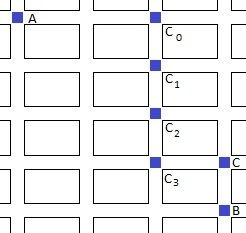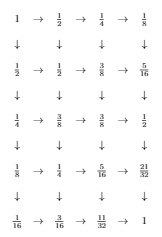# Getting from A to B via C

### Problem### Solution 1

The probability that the student passes through C is the sum of the probabilities that he passes through intersections $C_i,$ $i=0,1,2,3,$ and, at each, continues eastward:The number of ways of getting from A to $C_i$ equals $\displaystyle{2+i\choose 2}$ because each such path has two horizontal streets which may come in any order. The probability of taking each of the passes is $\displaystyle\left(\frac{1}{2}\right)^{3+i}$ due to the available number of choices on the way there. Thus, the total probability comes up to

$\displaystyle \sum_{i=0}^3{2+i\choose 2}\left(\frac{1}{2}\right)^{3+i}=\frac{1}{8}+\frac{3}{16}+\frac{6}{32}+\frac{10}{64}=\frac{21}{32}.$

### Remark

Just counting the number of ways from A to B and from A to C would not work. The numbers are $\displaystyle{6\choose 3}=20$ and $\displaystyle{7\choose 3}=35,$ allegedly giving the sought probability as $\displaystyle\frac{20}{35}=\frac{4}{7}.$ The reason that this does not work is that different pathways come with different probabilities such that simple comparison of their numbers is misleading.

### Solution 2

It is easy to construct a tree diagram to count probabilities of getting at every possible intersection:Summing up the probabilities we observe that for the nodes on the right edge going southward is the only chance and similarly for the bottom nodes. At all other nodes the chances are evenly split between the neighbors on the right and below.

### Solution 3The first location for the walker to reach the outside of $3\times 3$ subsquare must be one of the $6$ numbered spots. By symmetry, the probabilities on each side must sum to $\displaystyle \frac{1}{2}.$ Spots $1,2,3$ must pass through $C.$ From the other direction, the walker must pass through spot $6$ to reach $C.$

Thus the total probability is

$\displaystyle \frac{1}{2}+{5\choose 2}\cdot\left(\frac{1}{2}\right)^5\cdot \frac{1}{2} = \frac{21}{32}.$

### Solution 4

Solution via Markov chains: label each intersection with the probability that a path through it will pass through C before reaching B. Each intersection's probability is the average of its south and east neighbors. Working backwards from B, we find the probability for A is $\displaystyle \frac{21}{32}.$### Solution 5

Start with $32$ paths from $A,$ dividing paths into equals at each step and conserving the number of paths at each vertex. $21$ of the $32$ paths go through $C.$ Answer: $\displaystyle \frac{21}{32}:$### Solution 6

Key observation is that Pascal's triangle -- the number of ways to reach the intersection -- continues beyond the Eastern edge; everything that crosses that edge also gets to $C.$ So, $\displaystyle \frac{20+15+6+1}{2^6} = \frac{21}{32} = 0.65625.$### Solution 7

We introduce the following node (intersection) enumeration:

$\begin{array}{ccccc} 1&5&9&13&17\\ 2&6&10&14&18\\ 3&7&11&15&19\\ 4&8&12&16&20 \end{array}$

with the connectivity graph,so that the role of $C$ is taken up by the node number $16.$ Here are the probabilities of passing through each of the twenty nodes:### Acknowledgment

This is problem 25 from the 1982 AHSME. This is a borrowing from The Contest Problem Book IV by R. A. Artino, A. M. Caglione and N. Shell (MAA, 1982).

Solutions 3 is by Christopher D. Long; Solution 4 by Josh Jordan; Solution 5 is by Amit Itagi; Solution 6 is by Daren Chapin; Solution 7 is by N. N. Taleb.